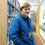Complex roots part 2 - Sum of the roots

$\sum_{x = 0}^{n - 1}{Z_x} = 0$

$\large Z_x = \sqrt[n]{z} = \sqrt[2n]{a^2 + b^2}\text{ cis}\left(\frac{\arctan{\frac{b}{a}}}{n} + \frac{2x\pi}{n}\right)$

Both of the above equations hold true as long as $\boxed{n \neq 0}$. But why does the top one hold true?

That's what we're going to be answering. So let's begin.

$\sum_{x = 0}^{n - 1}{Z_x} = Z_0 + Z_1 + \cdots + Z_{n - 1}$

$\sum_{x = 0}^{n - 1}{Z_x} = \sqrt[2n]{a^2 + b^2}\text{ cis}\left(\frac{\arctan{\frac{b}{a}}}{n}\right) + \sqrt[2n]{a^2 + b^2}\text{ cis}\left(\frac{\arctan{\frac{b}{a}}}{n} + \frac{2\pi}{n}\right) + \cdots + \sqrt[2n]{a^2 + b^2}\text{ cis}\left(\frac{\arctan{\frac{b}{a}}}{n} + \frac{2(n - 1)\pi}{n}\right)$

$\sum_{x = 0}^{n - 1}{Z_x} = \sqrt[2n]{a^2 + b^2}\left(\text{ cis}\left(\frac{\arctan{\frac{b}{a}}}{n}\right) + \text{ cis}\left(\frac{\arctan{\frac{b}{a}}}{n} + \frac{2\pi}{n}\right) + \cdots + \text{ cis}\left(\frac{\arctan{\frac{b}{a}}}{n} + \frac{(2n - 2)\pi}{n}\right)\right)$

$\text{cis}(a) + \text{cis}(b) + \text{cis}(c) + \cdots = \text{cis}(a)(1 + \text{cis}(b - a) + \text{cis}(c - a) + \cdots)$

$\sum_{x = 0}^{n - 1}{Z_x} = \sqrt[2n]{a^2 + b^2}\left(\text{ cis}\left(\frac{\arctan{\frac{b}{a}}}{n}\right)\left(1 + \text{cis}\left(\frac{2\pi}{n}\right) + \text{cis}\left(\frac{4\pi}{n}\right) + \cdots + \text{cis}\left(\frac{(2n - 2)\pi}{n}\right)\right)\right)$

$\text{cis}(nx) = (\text{cis}(x))^n$

$\sum_{x = 0}^{n - 1}{Z_x} = \sqrt[2n]{a^2 + b^2}\left(\text{ cis}\left(\frac{\arctan{\frac{b}{a}}}{n}\right)\left(1 + \text{cis}\left(\frac{2\pi}{n}\right) + \left(\text{cis}\left(\frac{2\pi}{n}\right)\right)^2 + \cdots + \left(\text{cis}\left(\frac{2\pi}{n}\right)\right)^{n - 1}\right)\right)$

$\text{Let } X = \text{cis}\left(\frac{2\pi}{n}\right)$

$\sum_{N = 0}^{n - 1}{X^N} = 1 + X + X^2 + \cdots + X^{n - 1}$

$\sum_{N = 0}^{n - 1}{X^N} = \frac{1 - X^{n}}{1 - X}$

$\sum_{x = 0}^{n - 1}{Z_x} = \sqrt[2n]{a^2 + b^2}\left(\text{ cis}\left(\frac{\arctan{\frac{b}{a}}}{n}\right)\frac{1 - X^{n}}{1 - X}\right)$

$\sum_{x = 0}^{n - 1}{Z_x} = \sqrt[2n]{a^2 + b^2}\left(\text{ cis}\left(\frac{\arctan{\frac{b}{a}}}{n}\right)\frac{1 - \left(\text{cis}\left(\frac{2\pi}{n}\right)\right)^{n}}{1 - \text{cis}\left(\frac{2\pi}{n}\right)}\right)$

$\sum_{x = 0}^{n - 1}{Z_x} = \sqrt[2n]{a^2 + b^2}\left(\text{ cis}\left(\frac{\arctan{\frac{b}{a}}}{n}\right)\frac{1 - \text{cis}(2\pi)}{1 - \text{cis}\left(\frac{2\pi}{n}\right)}\right)$

$\text{cis}(2\pi) = \cos(2\pi) + i\sin(2\pi) = 1 + 0i = 1$

$\sum_{x = 0}^{n - 1}{Z_x} = \sqrt[2n]{a^2 + b^2}\left(\text{ cis}\left(\frac{\arctan{\frac{b}{a}}}{n}\right)\frac{1 - 1}{1 - \text{cis}\left(\frac{2\pi}{n}\right)}\right)$

$\sum_{x = 0}^{n - 1}{Z_x} = \sqrt[2n]{a^2 + b^2}\left(\text{ cis}\left(\frac{\arctan{\frac{b}{a}}}{n}\right)\frac{0}{1 - \text{cis}\left(\frac{2\pi}{n}\right)}\right)$

$\sum_{x = 0}^{n - 1}{Z_x} = 0$

Hope you enjoyed the note.Note by Jack Rawlin
3 years, 7 months ago

This discussion board is a place to discuss our Daily Challenges and the math and science related to those challenges. Explanations are more than just a solution — they should explain the steps and thinking strategies that you used to obtain the solution. Comments should further the discussion of math and science.

When posting on Brilliant:

• Use the emojis to react to an explanation, whether you're congratulating a job well done , or just really confused .
• Ask specific questions about the challenge or the steps in somebody's explanation. Well-posed questions can add a lot to the discussion, but posting "I don't understand!" doesn't help anyone.
• Try to contribute something new to the discussion, whether it is an extension, generalization or other idea related to the challenge.
• Stay on topic — we're all here to learn more about math and science, not to hear about your favorite get-rich-quick scheme or current world events.

MarkdownAppears as
*italics* or _italics_ italics
**bold** or __bold__ bold

- bulleted
- list

• bulleted
• list

1. numbered
2. list

1. numbered
2. list
Note: you must add a full line of space before and after lists for them to show up correctly
paragraph 1

paragraph 2

paragraph 1

paragraph 2

> This is a quote
This is a quote
# I indented these lines
# 4 spaces, and now they show
# up as a code block.

print "hello world"
# I indented these lines
# 4 spaces, and now they show
# up as a code block.

print "hello world"
MathAppears as
Remember to wrap math in $$...$$ or $...$ to ensure proper formatting.
2 \times 3 $2 \times 3$
2^{34} $2^{34}$
a_{i-1} $a_{i-1}$
\frac{2}{3} $\frac{2}{3}$
\sqrt{2} $\sqrt{2}$
\sum_{i=1}^3 $\sum_{i=1}^3$
\sin \theta $\sin \theta$
\boxed{123} $\boxed{123}$